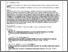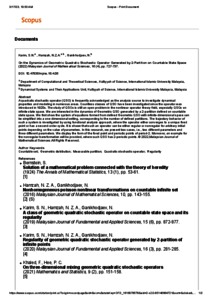# On the dynamics of geometric quadratic stochastic operator generated by 2-partition on countable state space

Karim, Siti Nurlaili and Hamzah, Nur Zatul Akmar and Ganikhodjaev, Nasir (2022) On the dynamics of geometric quadratic stochastic operator generated by 2-partition on countable state space. Malaysian Journal of Mathematical Sciences, 16 (4). pp. 727-737. ISSN 1823-8343Preview
PDF (SCOPUS) - Supplemental MaterialPDF (Article) - Published Version
Restricted to Repository staff only

## Abstract

A quadratic stochastic operator (QSO) is frequently acknowledged as the analysis source to investigate dynamical properties and modeling in numerous areas. Countless classes of QSO have been investigated since the operator was introduced in 1920s. The study of QSOs is still an open problem in the nonlinear operator theory field, especially QSOs on infinite state space. We are interested in the dynamics of Geometric QSO generated by a 2-partition defined on countable state space. We first show the system of equations formed from defined Geometric QSO with infinite-dimensional space can be simplified into a one-dimensional setting, corresponding to the number of defined partitions. The trajectory behavior of such a system is investigated by using functional analysis approach, where the operator either converges to a unique fixed point or has a second-order cycle. It is shown that such an operator can be either regular or nonregular for arbitrary initial points depending on the value of parameters. In this research, we present two cases, i.e., two different parameters and three different parameters. We display the form of the fixed point and periodic points of period-2. Moreover, an example for the nonregular transformation will be provided, where such QSO has 2-periodic points.

Item Type: Article (Journal) geometric distribution; quadratic stochastic operator; measurable partition; countable set; regularity. Q Science > QA Mathematics Kulliyyah of Science > Department of Computational and Theoretical SciencesKulliyyah of Science Nur Zatul Akmar Hamzah 17 Mar 2023 10:53 17 Mar 2023 10:54 http://irep.iium.edu.my/id/eprint/104084View Item